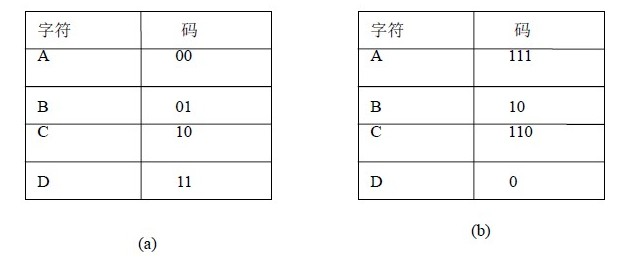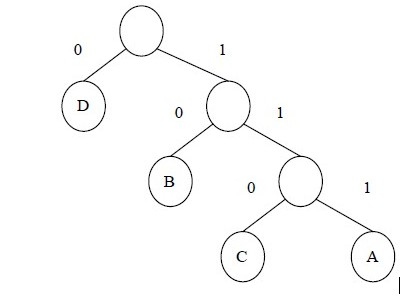1、那么什么是权值？什么是路径长度？什么是带权路径长度呢？

2、哈夫曼树的构造过程。（结合图例）

(1) 将w1、w2、…，wn看成是有n 棵树的森林(每棵树仅有一个结点)；

(2) 在森林中选出两个根结点的权值最小的树合并，作为一棵新树的左、右子树，且新树的根结点权值为其左、右子树根结点权值之和；

(3)从森林中删除选取的两棵树，并将新树加入森林；

(4)重复(2)、(3)步，直到森林中只剩一棵树为止，该树即为所求得的哈夫曼树

3、哈夫曼树的应用：哈夫曼编码（前缀编码）4、哈夫曼树的实现

public class Node
{
char c; //存储的数据，为一个字符
private double weight; //结点权值
private int lChild; //左孩子结点
private int rChild; //右孩子结点
private int parent; //父结点
//结点权值属性
public double Weight
{
get
{
return weight;
}
set
{
weight = value;
}
}
//左孩子结点属性
public int LChild
{
get
{
return lChild;
}
set
{
lChild = value;
}
}
//右孩子结点属性
public int RChild
{
get
{
return rChild;
}
set
{
rChild = value;
}
}
//父结点属性
public int Parent
{
get
{
return parent;
}
set
{
parent = value;
}
}
//构造器
public Node()
{
weight = 0;
lChild = -1;
rChild = -1;
parent = -1;
}
//构造器
public Node(double weitht)
{
this.weight = weitht;
lChild = -1;
rChild = -1;
parent = -1;
}

//构造器
public Node(int w, int lc, int rc, int p)
{
weight = w;
lChild = lc;
rChild = rc;
parent = p;
}
}

public class HuffmanTree
{
private Node[] data; //结点数组
private int leafNum; //叶子结点数目
//索引器
public Node this[int index]
{
get
{
return data[index];
}
set
{
data[index] = value;
}
}
//叶子结点数目属性
public int LeafNum
{
get
{
return leafNum;
}
set
{
leafNum = value;
}
}
//构造器
public HuffmanTree(int n)
{
data = new Node[2 * n - 1];
leafNum = n;
}
//创建哈夫曼树
public void Create(Node[] datain)
{
double minL, minR;
minL = minR = double.MaxValue;
int lchild, rchild;
lchild = rchild = -1;

int length = data.Length;
//初始化哈夫曼树
for (int i = 0; i < length; i++)
data[i] = new Node();
for (int i = 0; i < datain.Length; i++)
data[i] = datain[i];

//处理n个叶子结点，建立哈夫曼树
for (int i = leafNum; i < length; i++)
{
//在全部结点中找权值最小的两个结点
for (int j = 0; j < i; j++)
{
if (data[j].Parent == -1)
{
if (data[j].Weight < minL)
{
minR = minL;
minL = data[j].Weight;
rchild = lchild;
lchild = j;
}
else if (data[j].Weight < minR)
{
minR = data[j].Weight;
rchild = j;
}
}
}
data[lchild].Parent = data[rchild].Parent = i;
data[i].Weight = minL + minR;
data[i].LChild = lchild;
data[i].RChild = rchild;
}
}
}

class Program
{
static void Main(string[] args)
{
HuffmanTree tree = new HuffmanTree(5);
Node[] nodes = new Node[] { new Node(1), new Node(2), new Node(2.5d), new Node(3), new Node(2.6d) };
tree.Create(nodes);

}
}

/////////////////////////////节选自网络上的资料、

posted on 2011-10-02 17:04 Yu_ 阅读(2632) 评论(1)  编辑 收藏 引用 所属分类: 数据结构FeedBack:
# re: 哈夫曼树
2013-07-07 04:01 | johan